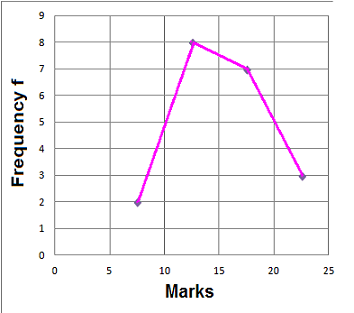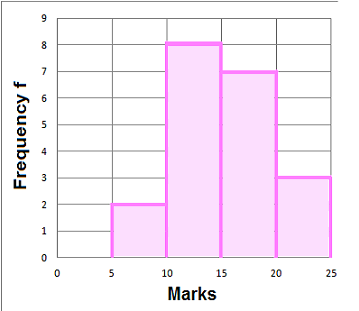### Frequency Diagrams and Frequency Polygons

When data is grouped with equal intervals, work out the midpoint.

A frequency diagram can be drawn then with the midpoint along the horizontal (x-axis) and the frequency along the vertical (y-axis)

A frequency polygon is formed by joining the midpoints of the tops of the bars in a frequency diagram.

#### Example 1.   Draw a frequency diagram and frequency polygon of the grouped data:

 Marks Frequency f 5≤M≤10 2 10≤M≤15 8 15≤M≤20 7 20≤M≤25 3

(a) For each range of Marks work out the midpoint x

 Marks f Midpoint x 5≤M≤10 2 7.5 10≤M≤15 8 12.5 15≤M≤20 7 17.5 20≤M≤25 3 22.5

(b) Frequency diagram(c) Frequency polygonto: